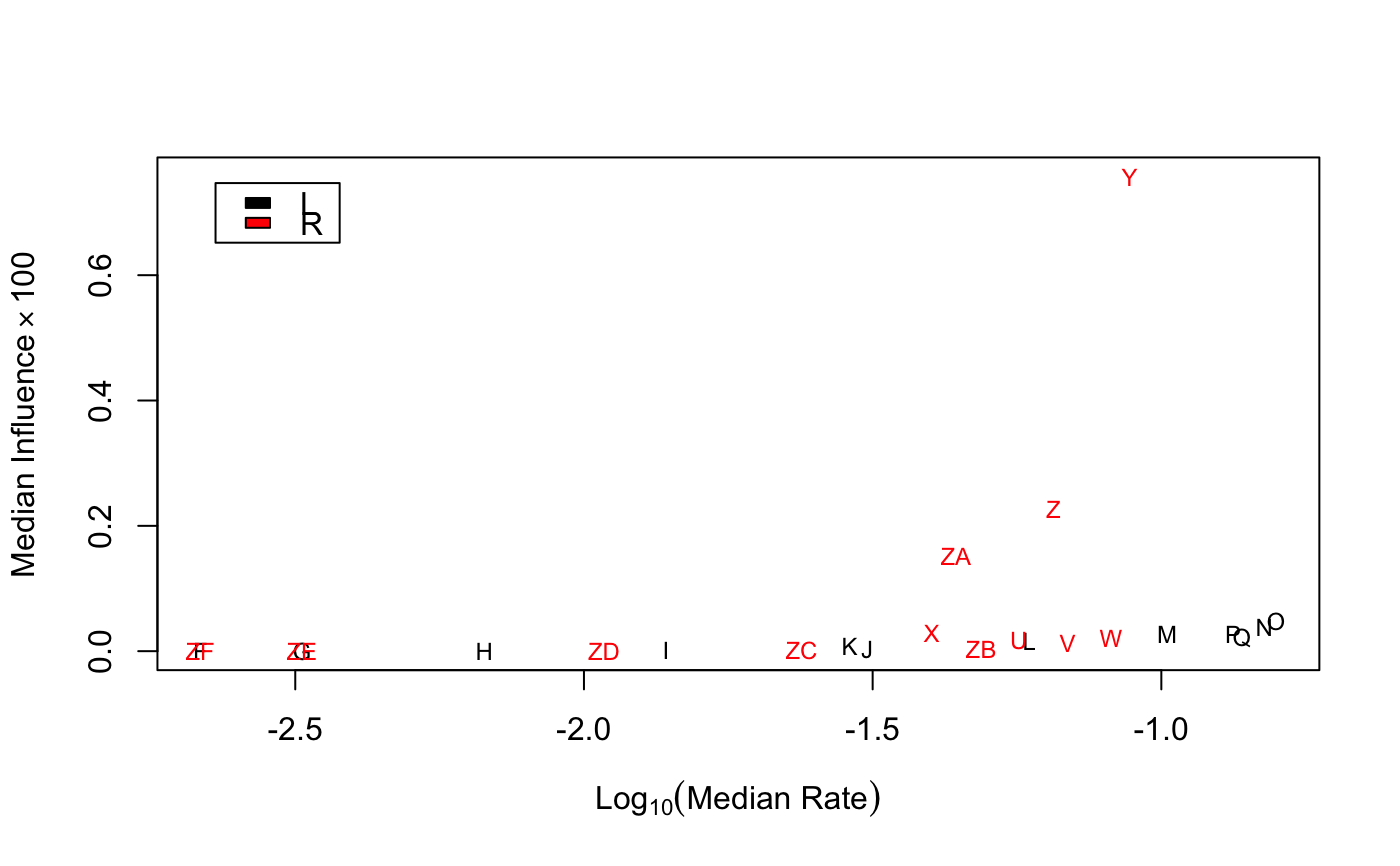Plot the results of a fitted scaling model, from (e.g.) a predicted textmodel_affinity model.

textplot_influence(x, n = 30, ...)

## Arguments

x the object output from influence() run on the fitted or predicted scaling model object to be plotted the number of features whose influence will be plotted additional arguments passed to plot

textmodel_affinity
influence.predict.textmodel_affinity
tmod <- textmodel_affinity(data_dfm_lbgexample, y = c("L", NA, NA, NA, "R", NA))
textplot_influence(influence(pred))